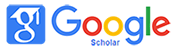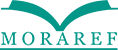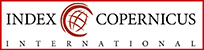### INFLUENCE OF CROSS-LINE TECHNIQUE TO ABILITY OF MATHEMATICAL REPRESENTATION ON CONTENT MULTIPLICATION OF CLASS III SD AL-ZAHRA INDONESIA

#### Abstract

This study aims to determine the effect of cross-line techniques on the ability of the student representation on the material multiplication in Al-Zahra Indonesia Elementary third grade second semester of the academic year 2017/2018. Cross-line technique is one technique that can facilitate students in operating multiplication on learning mathematics. The method used in this research is quasi experiment with Design Nonequivalent Contol Group Design. The sample used in this study consisted of 23 experimental class and control class which consisted of 22 students. The instrument used in this research is a test of mathematical representation ability in the form of description problem. The results showed that the average score of students taught by using cross-line technique is higher than the students taught without using cross-line technique that is with the value of 80,57> 70,05. The data analysis of the two classes was calculated using statistical analysis test on the final test (posttest). Based on the result of data analysis, there are significant differences between the two classes which are seen from the test result of Mann-Whitney U test hypothesis on post test data (0,048<0,05). In addition, based on the results of the calculation effect test (effect size) that has been done using the formula Cohen's got the value of the effect size of 0.561. The value of the influence test obtained interpreted that cross-line techniques have influence in the medium category. Thus, this indicates that there is an influence of the use of techniques that are cross-line to the ability of the mathematical representation in third grade elementary multiplication material of Al-Zahra Indonesia

#### Keywords

Cross-line Technique; Mathematical Representation Ability; Quasi Experiments.

#### Full Text:

PDF

DOI: http://dx.doi.org/10.32934/jmie.v3i1.90

### Refbacks

• There are currently no refbacks.